# C133 – Density Computation – Micro Motion (Solartron)

## Description

This calculation returns a corrected density value and a corrected time period based on options set by the user.

Solartron 7812 Manual

Solartron 7835, 7845/46/47 liquid density transducer technical manual

Schlumberger Type 7915 Flow Computer Operating Manual Part 79155001 Issue A 1991

Kelton calculation reference C133

KIMS calculation reference K149

The FLOCALC.net version of this calculation reference F012 has been replaced by the following calculations:

F081: Gas Densitometer Computation – Micro Motion

F082: Liquid Densitometer Computation – Micro Motion

## Options

### Fluid

Select whether a liquid or gas densitometer is being used

• Gas
• Liquid

### Correct for

Select the corrections to apply

For a gas

• Temperature
• Velocity of Sound
• Upstream Conditions

For a liquid

• Temperature
• Pressure

### Revised Pressure Constants

Select to use the new pressure calibration equation for liquid densitometers

• Use K20C and K21C

### Temperature-pressure coupling

Select this to apply the temperature-pressure coupling correction for liquid densitometers

• Apply

### Calibration certificate units

Select the unit that is used for pressure in the densitometer calibration certificate. This option is available for liquid densitometers. Note that the pressure inputs for this calculation can be entered in any units but the calibration certificate units are important as although the K coefficients are unitless on the certificate they are not dimensionless and do have effective units e.g K19 has dimensions density/temperature, K21A has dimensions density/pressure, K21B has units density/pressure2 etc.

• bar and °C
• psi and °F
• kPa and °C

### VoS Method

Select velocity of sound method

• P/D Method
• User Gas Method

### Calibration Gas

This option allows the user to specify which calibration gas is being used.

If ‘User Gas Method’ is selected

• Nitrogen
• Argon
• Other

If ‘P/D Method’ is selected

• Nitrogen (0-100kg/m3)
• Nitrogen (0-400kg/m3)
• Methane (0-250kg/m3)
• Argon (0-400kg/m3)

If ‘User Gas Method’ is selected the following options apply

### Calbration Gas Constant

If ‘Other’ is selected from ‘Calibration Gas’ specify which way the calibration gas constant is being entered.

• User Entered
• Calculated from ratio (G)
• Calculated from Specific Gravity (SG)

### Measured Gas Ratio

• User entered
• Calculated

If ‘P/D Method’ is selected the following options apply

### Transducer Type

If the velocity of sound option ‘P/D method’ is selected you are given the option to either select a transducer model or select ‘other’ in which case you will be able to enter the velocity of sound constant as an input.

### VOS of Measured Gas

• User entered
• Calculated

If ‘Calculated’ is selected a gas composition needs to be input to calculate the velocity of sound.

### VOS of Calibration Gas

• User entered
• Calculated

If ‘Calculated’ is selected then velocity of sound is determined form the calibration gas option.

## Calculations

### Density Calculation

The uncorrected density ρ is calculated by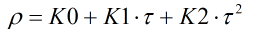Where K0 = Densitometer constant obtained from the calibration certificate K1 = Densitometer constant obtained from the calibration certificate K2 = Densitometer constant obtained from the calibration certificate τ = Time period in µs

### Temperature Correction

The temperature corrected density ρt is calculated by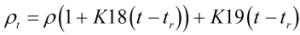Where ρ = Uncorrected density K18 = Densitometer constant obtained from the calibration certificate K19 = Densitometer constant obtained from the calibration certificate t = Transducer temperature tr = Reference temperature

### Pressure Correction

If the pressure corrected density ρc is to be calculated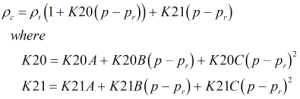Where ρt = Temperature corrected density K20A = Densitometer constant obtained from the calibration certificate K20B = Densitometer constant obtained from the calibration certificate K20C = Densitometer constant obtained from the calibration certificate K21A = Densitometer constant obtained from the calibration certificate K21B = Densitometer constant obtained from the calibration certificate K21C = Densitometer constant obtained from the calibration certificate p = Transducer pressure pr = Reference pressure

Note that the K20C and K21C terms are only applied for newer densitometers, for older models these additional terms are not included in the pressure correction calculation.

### Temperature Pressure Coupling Correction

The temperature pressure coupling correction is used to correct the density according to the formula: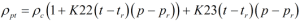Where ρc = Pressure corrected density K22 = Densitometer constant obtained from the calibration certificate K23 = Densitometer constant obtained from the calibration certificate t = Transducer temperature tr = Reference temperature p = Transducer pressure pr = Reference pressure

### VoS – P/D Method

The pressure/density method of determining the velocity of sound correction is calculated by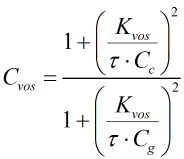Where Cvos = Velocity of sound correction factor K = Velocity of sound constant for the density sensor τ = Time period in µs Cc = Velocity of sound of calibration gas Cg = Velocity of sound of measured gas

### Velocity of sound of measured and calibration gas

For the measured gas this is calculated in the Solartron 7915 Flow Computer by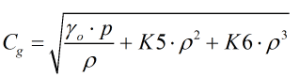Where γo = The ratio of specific heat for the composition K5 = Constant for the composition calculated from table 51 K6 = Constant for the composition calculated from table 51

For the calibration gas this is calculated in the Solartron 7915 Flow Computer by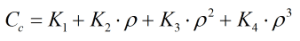Where Kn = Constants for the calibration gas

### VoS – User Gas Method

The user method for determining the velocity of sound correction is calculated by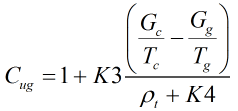Where Cug = The user gas correction factor K3 = A constant for the density sensor K4 = A constant for the density sensor Tc = The temperature of the calibration gas Tg = The temperature of the measured gas Gc = The Specific Gravity / Ratio of specific heats of calibration gas assumed to be 0.6908264 for Nitrogen and 0.8224192 for Argon Gg = The Specific Gravity / Ratio of specific heats of measured gas

### Time period calculation

The corrected time period τc can be calculated by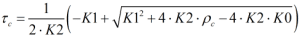Where K0 = Densitometer constant obtained from the calibration certificate K1 = Densitometer constant obtained from the calibration certificate K2 = Densitometer constant obtained from the calibration certificate ρc = The corrected density

Back